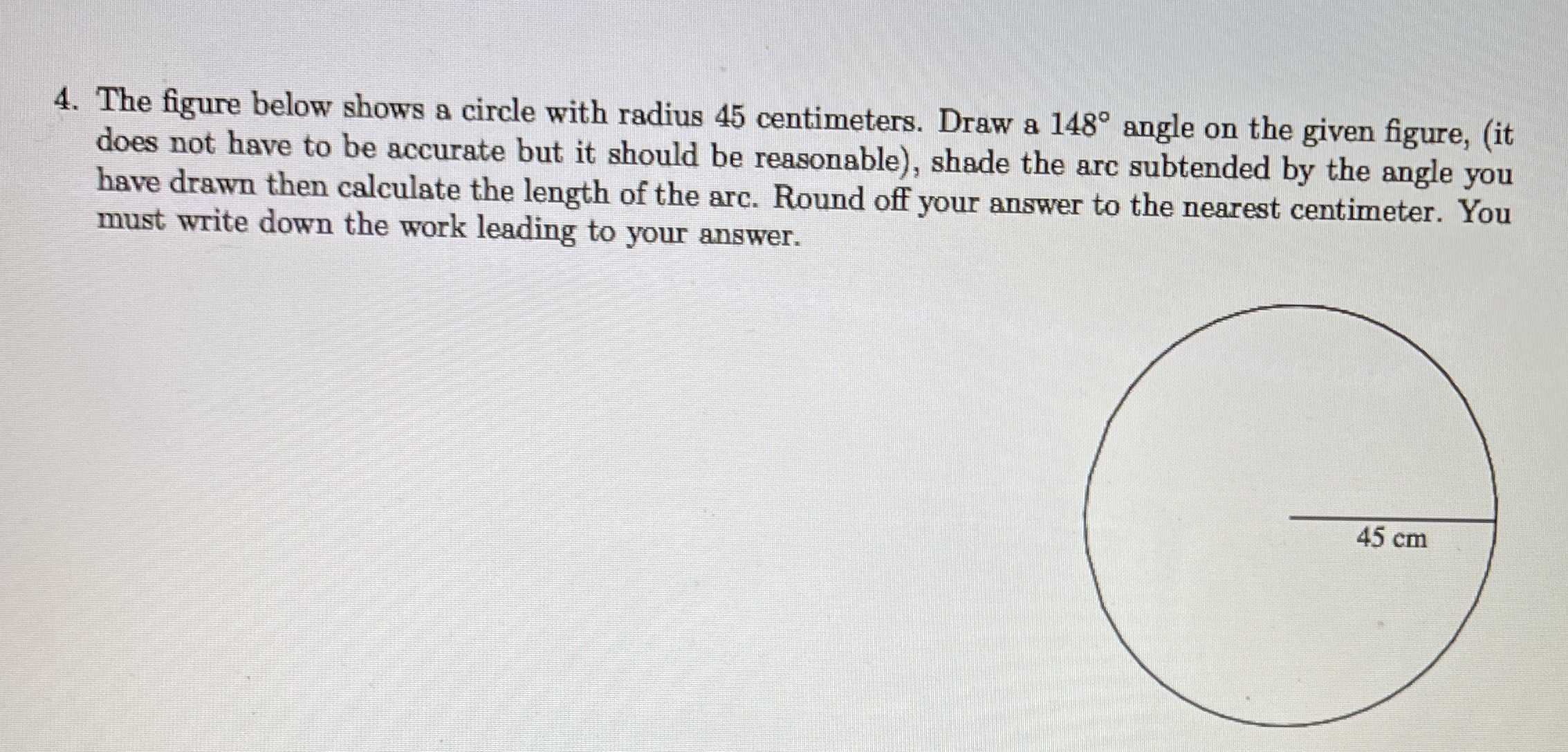### ¿Todavía tienes preguntas de matemáticas?

Pregunte a nuestros tutores expertos
Algebra
Pregunta4. The figure below shows a circle with radius $$45$$ centimeters. Draw a $$148 ^ { \circ }$$ angle on the given figure, (it does not have to be accurate but it should be reasonable), shade the arc subtended by the angle you have drawn then calculate the length of the arc. Round off your answer to the nearest centimeter. You must write down the work leading to your answer.